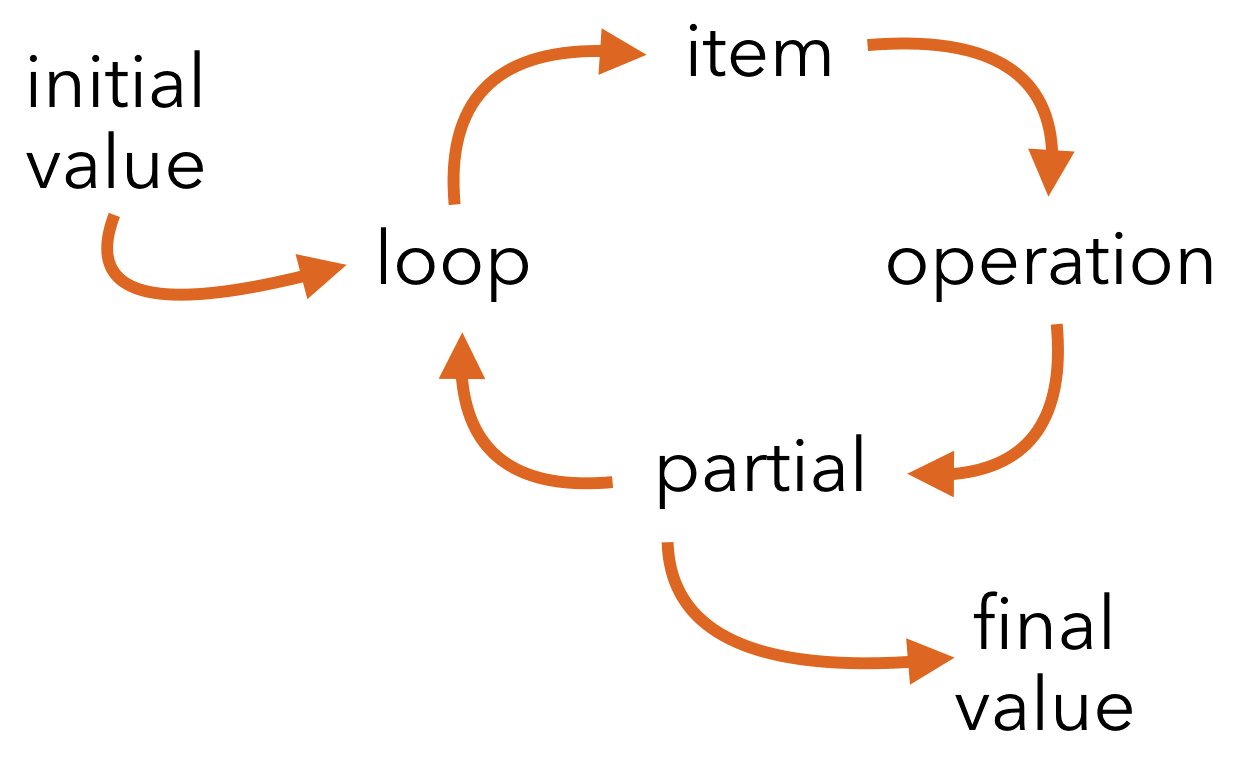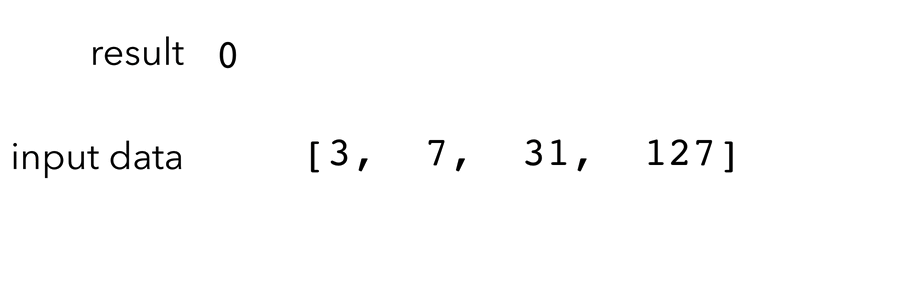Reduce

Map and filter get all the glory, but reduce is the quiet workhorse.

5 April 2017 ∙ Swift Language ∙ written by

Filter, reduce, and map:
Collections transformed at a tap.
These functional friends,
with immutable inputs, no trap.

Map and filter get a lot of attention, but I say reduce is the important one. Or if you’re interested in efficiency, how about this: you can build map and filter using reduce. If you’re going to learn about just one, pick reduce.

Functional Friends

Map has the useful behavior of performing some operation on every item in the sequence and collecting the results.

``````let words = ["hello", "there"]
let shoutyWords = words.map { \$0.uppercased() }
// ["HELLO", "THERE"]
``````

The input to `map` is a sequence of size n, and the output will also be of size n.

Next, filter lets you decide which items to keep (and which to discard) from a sequence.

``````let numbers = 0...10
let evenNumbers = numbers.filter { \$0 % 2 == 0 }
// [0, 2, 4, 6, 8, 10]
``````

The output size will be somewhere between 0 and n, depending on how many things get filtered.

What of reduce?

Reducing Reduce

Like the other two operations, `reduce` takes a sequence as input but its output is more generic: it can be anything you want!

For every turn of the loop, `reduce` passes the previous output along with the current item. That means you get a some state preserved between iterations and can build up the output however you need it.

For the first time through, you need to provide an initial starter “result” value. That means `reduce` takes two arguments:

1. The initial result value.
2. A closure that produces the next partial result.

Reduce will pass in the partial result and next value as arguments to the closure.Using Reduce

The textbook example of `reduce` is finding the sum of numbers:

``````let primes = [3, 7, 31, 127]
let sum = primes.reduce(0) { (result, item) in
return result + item
}
``````

The first time through, `reduce` will call `closure(0, 3)` where 0 is the initial value and 3 is the first item. The result is now 0 + 3, or 3.

The next time through the loop, `reduce` will call `closure(3, 7)` where 3 is the result from last time and 7 is the next item. And so on.At the end, we’ll have turned an array `[Int]` into the final result of type `Int`.

Building Reduce

Let’s have a look at how `reduce` is built in the standard library. As usual, the code will be inline below and you can see the code yourself on GitHub in SequenceAlgorithms.swift.

It’s pretty short once you get through the slightly gnarly function signature:

``````public func reduce<Result>(
_ initialResult: Result,
_ nextPartialResult:
(_ partialResult: Result, Iterator.Element) throws -> Result
) rethrows -> Result {
``````

There are a lot of `Result` types here — that’s the generic type describing what the final return value will be. This can be different from `Iterator.Element`, which is the type of the thing in the sequence.

The `initialResult` parameter is of type `Result`, and needs to be passed into the function as the first argument.

The second parameter is the closure that runs for each value in the sequence. The closure itself gets passed two arguments:

1. The result returned from the previous iteration.
2. The next item in the sequence.

Finally, the `throws` and `rethrows` keywords allow for closures that throw errors; these will be rethrown up the chain to the original caller if needed.

Accumulating Results

The `accumulator` variable will hold the cumulative result as we iterate through the sequence.

``````var accumulator = initialResult
``````

We’ll start out with the initial result passed into the function.

Next up is the big iteration step:

``````for element in self {
accumulator = try nextPartialResult(accumulator, element)
}
``````

The accumulator gets replaced each time through the loop with the new partial value returned from the closure. Again, the closure here takes the previous result and the next element.

Just one more line to go:

``````return accumulator
``````

By the end, all that’s left to do is return the last result.

Building Map

How might you build `map` with reduce? For simplicity, we’ll work with arrays rather than sequences.

Let’s think about `map` in terms of how `reduce` works:

• The result type will be an array
• The initial value should be an empty array
• For each turn of the loop, add an item to the array
``````extension Array {
func mapUsingReduce<OutElement>(
_ closure: (_ item: Iterator.Element) -> OutElement)
-> [OutElement] {
return self.reduce([]) { (partial, item) in
return partial + [closure(item)]
}
}
}
``````

The generic parameter `OutElement` is the type of element in the output array. The method takes a closure to perform the transform step of converting an `Iterator.Element` into an `OutElement`.

The `reduce` call is as expected here: start with an empty array, and keep adding to the accumulated array each time through the loop.

Can you think of how to write `filter` using `reduce`? How about `sort`? What other uses can you think of? How efficient is doing `map` this way vs actual `map`?

The Closing Brace

This is one of those times where learning how to use a hammer means every problem starts looking like a nail (to wrangle another turn of phrase). Whenever I need some kind of operation on an array, I start thinking of how I might do it with `reduce`.

That doesn’t mean I actually use `reduce` though — it isn’t the best solution for every problem! What I like about it is it makes me think through the basics: initial value, an incremental step, and how to slowly build up a return value.

Download Reduce.playground to check out the code and play around with it yourself.

`}`